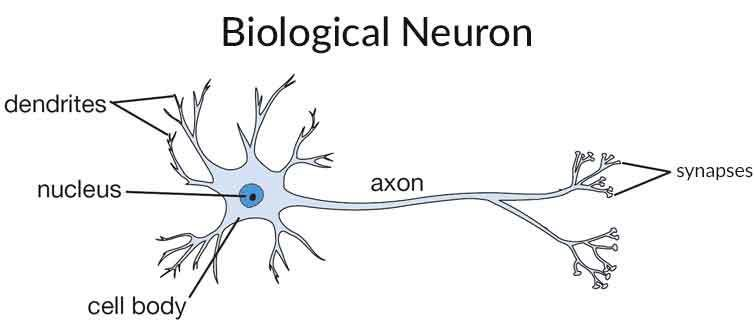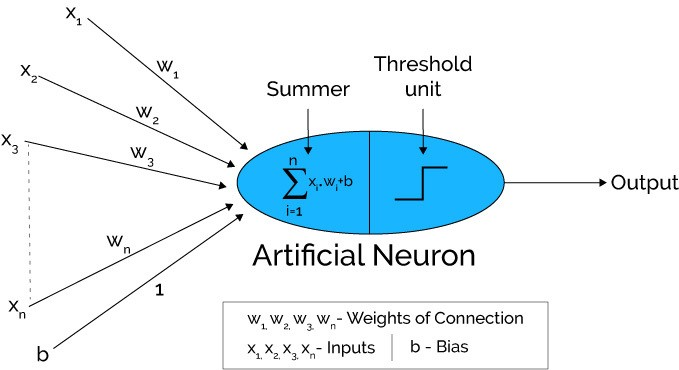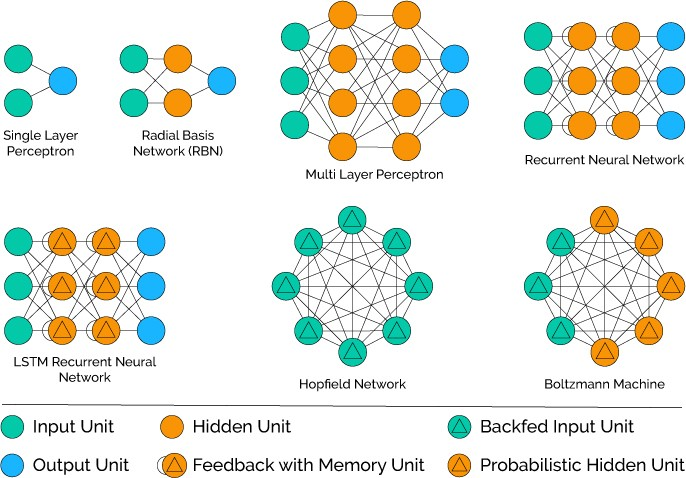# 从锅炉工到AI专家(5)

TensorFlow实务

#### 图像识别基本原理#### 神经网络

“神经网络”的诞生是自然选择的结果，人脑就是由无数个神经元组成的，有资料说大概接近900亿个，是天文数字的级别。这些神经网络的传导和反射支撑着现代人类所有的智力和行为。#### 神经网络图像识别源码

``````#!/usr/bin/env python
# -*- coding=UTF-8 -*-

import input_data
mnist = input_data.read_data_sets('MNIST_data', one_hot=True)

import tensorflow as tf
sess = tf.InteractiveSession()

#对W/b做初始化有利于防止算法陷入局部最优解，
#文档上讲是为了打破对称性和防止0梯度及神经元节点恒为0等问题，数学原理是类似问题
#这两个初始化单独定义成子程序是因为多层神经网络会有多次调用
def weight_variable(shape):
#填充“权重”矩阵，其中的元素符合截断正态分布
#可以有参数mean表示指定均值及stddev指定标准差
initial = tf.truncated_normal(shape, stddev=0.1)
return tf.Variable(initial)
def bias_variable(shape):
#用0.1常量填充“偏移量”矩阵
initial = tf.constant(0.1, shape=shape)
return tf.Variable(initial)

#定义占位符，相当于tensorFlow的运行参数，
#x是输入的图片矩阵，y_是给定的标注标签，有标注一定是监督学习
x = tf.placeholder("float", shape=[None, 784])
y_ = tf.placeholder("float", shape=[None, 10])

#定义输入层神经网络，有784个节点，1024个输出，
#输出的数量是自己定义的，要跟第二层节点的数量吻合
W1 = weight_variable([784, 1024])
b1 = bias_variable()
#使用relu算法的激活函数，后面的公式跟前一个例子相同
h1 = tf.nn.relu(tf.matmul(x, W1) + b1)

#定义第二层（隐藏层）网络，1024输入，512输出
W2 = weight_variable([1024, 512])
b2 = bias_variable()
h2 = tf.nn.relu(tf.matmul(h1, W2) + b2)

#定义第三层（输出层），512输入，10输出，10也是我们希望的分类数量
W3 = weight_variable([512, 10])
b3 = bias_variable()
#最后一层的输出同样用softmax分类（也算是激活函数吧）
y3=tf.nn.softmax(tf.matmul(h2, W3) + b3)

#交叉熵代价函数
cross_entropy = -tf.reduce_sum(y_*tf.log(y3))
#计算正确率以评估效果
correct_prediction = tf.equal(tf.argmax(y3,1), tf.argmax(y_,1))
accuracy = tf.reduce_mean(tf.cast(correct_prediction, "float"))
#tf初始化及所有变量初始化
sess.run(tf.global_variables_initializer())
#进行20000步的训练
for i in range(20000):
#每批数据50组
batch = mnist.train.next_batch(50)
#每100步进行一次正确率计算并显示中间结果
if i%100 == 0:
train_accuracy = accuracy.eval(feed_dict={
x:batch, y_: batch})
print "step %d, training accuracy %g"%(i, train_accuracy)
#使用数据集进行训练
train_step.run(feed_dict={x: batch, y_: batch})

#完成模型训练给出最终的评估结果
print "test accuracy %g"%accuracy.eval(feed_dict={
x: mnist.test.images, y_: mnist.test.labels})
``````

(待续…)

#### 引文及参考